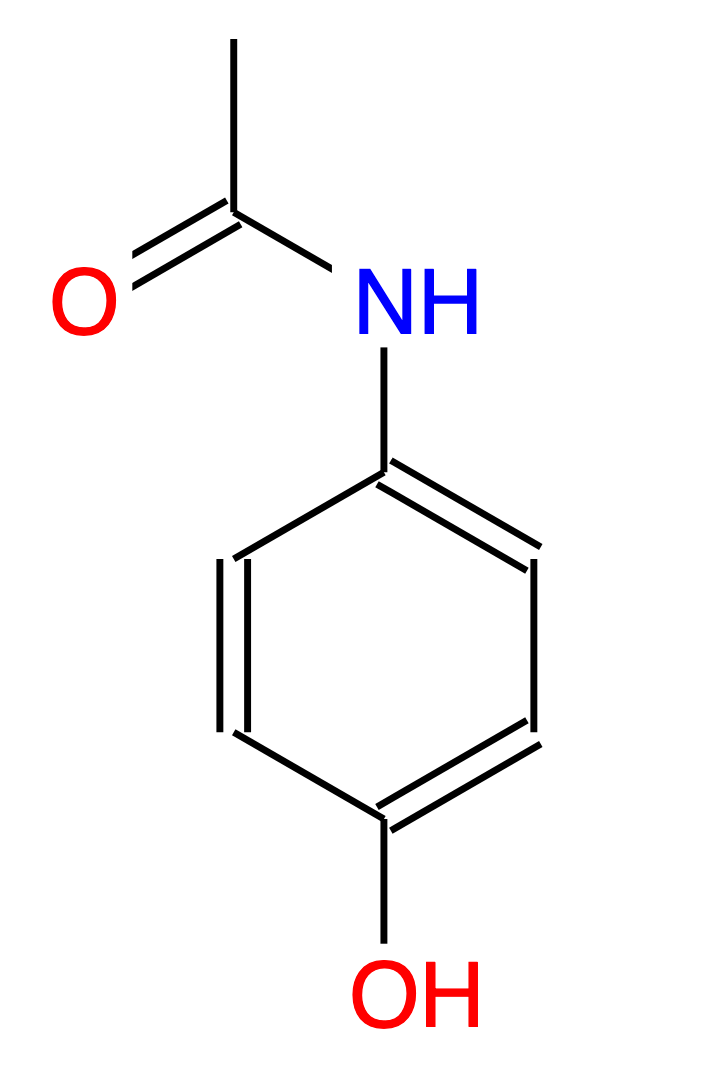# Page History

## Key

• This line was removed.
• Formatting was changed.

The Multilevel Neighbourhoods of Atoms (MNA) descriptors represent a molecule as a set of character strings.An example of MNA descriptors is given here below for Paracetamol:

HC

HN

HO

CHHHC

CHCC

CCCN

CCCO

CCNO

NHCC

OHC

OC

C(C(CC-H)C(CC-H)-N(C-H-C))

C(C(CC-H)C(CC-H)-O(C-H))

C(C(CC-H)C(CC-N)-H(C))

C(C(CC-H)C(CC-O)-H(C))

-H(C(CC-H))

-H(-C(-H-H-H-C))

-H(-N(C-H-C))

-H(-O(C-H))

-C(-H(-C)-H(-C)-H(-C)-C(-C-N-O))

-C(-C(-H-H-H-C)-N(C-H-C)-O(-C))

-N(C(CC-N)-H(-N)-C(-C-N-O))

-O(C(CC-O)-H(-O))

-O(-C(-C-N-O))An important feature of the MNA descriptors is that they are constructed directly using the structural formula rather than a prescribed list of structural fragments. Yet another feature of these descriptors consists in that they retain the integrity of structural fragments in the sense that for each MNA descriptor the researcher can draw the corresponding structural fragment provided some skill.

...